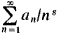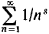# Dirichlet Series

(redirected from Abscissa of convergence)

## Dirichlet series

[‚dē·rē′klā ‚sir·ēz]
(mathematics)
A series whose n th term is a complex number divided by n to the z th power.

## Dirichlet Series

(named for P. G. L. Dirichlet), series of the formwhere the an are constants and s = σ + it is a complex variable. For example, the seriesrepresents the zeta function for σ > 1. The theory of Dirichlet series originally arose under the strong influence of analytic number theory. Eventually it developed into an extensive branch in the theory of analytic functions.

Site: Follow: Share:
Open / Close